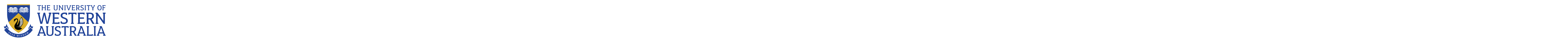School of Mathematics and Statistics

# School of Mathematics and Statistics

Research being undertaken by our current postgraduates:

Sylvia Morris

Aug 2011

Aug 2013

### Sylvia Morris

#### Thesis

Symplectic translation planes, pseudo-ovals, and maximal 4-arcs

#### Summary

This thesis is comprised of the study of three distinct problems: the construction of symplectic translation planes, the characterisation of pseudo-ovals, and the classification of maximal arcs.

Non-Desarguesian planes are the only finite projective spaces not to be governed by a finite field and, as such, hold a certain interest for finite geometers. In the ‘50s and ‘60s, Andr ́e, Bruck and Bose, and Segre developed a method — now commonly referred to as the Andr ́e/Bruck-Bose construction — to create translation planes, a subset of projective planes that includes many non-Desarguesian planes, from spreads of higher dimensional projective spaces. In Chapter 4, we outline previous computational work in this area, the linear programming methods we have used, and present eight new examples of translation planes. These examples correspond to spreads of W(5, 5) and W(5, 7). Appendix A contains the details required to reconstruct each of these spreads.

Examples of pseudo-ovals can be constructed from ovals, using field reduction, and the existence of other types of pseudo-oval is unknown. Pseudo-ovals are represented, via geometric passages, as ovals in translation planes, as elements of translation generalised quadrangles, and, in some cases, as special sets in the Hermitian space H(3,q2). In Chapter 5 we go through relevant theorems in each situation and summarise the current status of the problem.

The Mathon maximal arcs, generalised from the Denniston maximal arcs, are each formed by taking a union of regular hyperovals on a common nucleus. In Chapter 6 we prove that all maximal 4- arcs, in the Desarguesian plane PG(2, q), that are a union of regular hyperovals or that contain two conics that share exactly one point, are Mathon maximal 4-arcs. This is joint work with Nicola Durante from the Universit ́a degli Studi di Napoli Federico II.

#### Why my research is important

Information gained in this area would give rules and reasons as to when field reduction could be used to create new geometrical objects and when it is likely to aid in solving conjectures. In itself this is interesting because it would give us a deeper understanding of how projective and polar spaces of different order are linked. Looking outside its intrinsic value, knowledge of when to apply field reduction would also prove to be widely applicable within finite geometry.

### Statistics clinic

Assistance in statistics is available for Postgraduates students by research at the UWA Centre for Applied Statistics.

# The University of Western Australia

## University information

CRICOS Code: 00126G